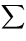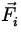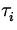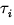# Another Condition for Equilibrium

For a body to be in equilibrium, Newton's laws require the sum of the forces to be zero:= 0 (ForceBalance). (3)

If the body is an extended object, then the forces don't necessarily act at the same point. Thus, even if the vector sum of the forces is zero, so that there is no net linear acceleration of the object, it is possible that the net torque is not zero. The second condition for equilibrium that needs to be imposed is therefore:= 0 (TorqueBalance) (4)

where the torquescan be calculated about any axis. One can therefore choose the most convenient axis for the given problem.